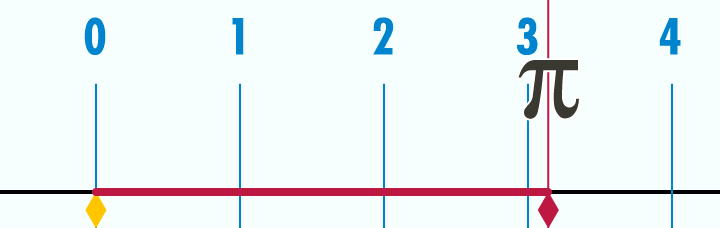## How to Calculate the Circumference of a Circle

In the previous post, we have learned about the basic terminologies about circles. We continue this series by understanding the meaning of circumference of a circle. The circumference of a circle is basically the distance around the circle itself. If you want to find the circumference of a can, for example, you can get a measuring tape and wrap around it.

The animation below shows, the meaning of circumference. As we can see, the circle with diameter 1 has circumference$\pi$ or approximately$3.14$.Note: If you want to know where$\pi$ came from, read Calculating the Value of Pi.

Example 1

What is the perimeter of a circle with diameter 1 unit?  » Read more